判别模型(Discriminative model)和生成模型(Generative model)

1.1 判别模型和生成模型的区别

p(y|x)=p(x|y)p(y)p(x)

argmaxyp(y|x)=argmaxyp(x|y)p(y)p(x)=argmaxyp(x|y)p(y)

1.2 高斯生成模型
1.2.1高斯生成模型概念

yBernoulli(ϕ)

x|y=0N(μ0,Σ)

x|y=1N(μ1,Σ)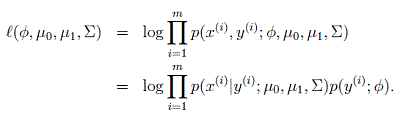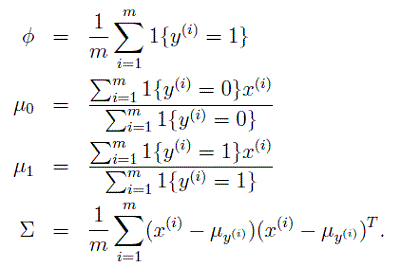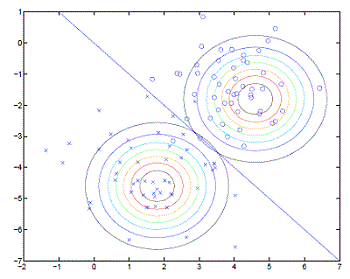1.2.2高斯生成模型与逻辑回归的关系

p(y=1|x;ϕ,μ0,μ1,Σ)=11+exp(θTx)

1.3朴素贝叶斯
1.3.1 朴素贝叶斯基本概念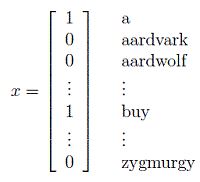p(x|y)=p(x1,...,x500000|y)=p(x1|y)p(x2|y,x1)p(x3|y,x1,x2)...p(x500000|y,x1,...,x499999)=p(x1|y)p(x2|y)p(x3|y)...p(x500000|y)=i=1np(xi|y)

L(ϕi|y=1,ϕi|y=0,ϕy)=i=1mp(x(i),y(i))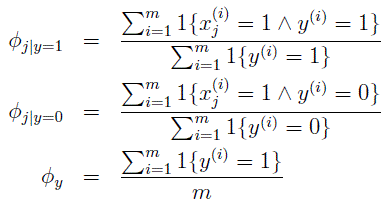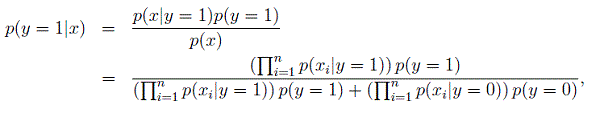1.设特征x={x1,x2,...,xm}$x=\{x_1,x_2,...,x_m\}$，每一个分量表示特征的一个属性维度
2.设有类别集合C=y1,y2,...,yn$C={y_1,y_2,...,y_n}$
3.分别计算特征x$x$属于每一个类别的概率大小，p(y1|x),p(y2|x),...,p(yn|x)$p(y_1|x),p(y_2|x),...,p(y_n|x)$

4.特征x$x$类别为最大概率所对应的类别，argmaxyip(yi|x)$argmax_{y_i}{p(y_i|x)}$

1.3.2 朴素贝叶斯例子

1.选取特征。

x1:x10.05,0.05<x1<0.2,x10.2$x_1:{x_1\le0.05,0.05x2:x20.1,0.1<x2<0.8,x20.8$x_2:x_2\le0.1, 0.1x3x3=0,x3=1$x_3：{x_3=0（不是）,x_3=1（是）}$

2.选取训练样本

3.计算类别概率p(y)$p(y)$
p(y=0)=8900/10000=0.89,p(y=1)=1100/10000=0.11$p(y=0)=8900/10000=0.89,p(y=1)=1100/10000=0.11$

4.计算每个类别的各个特征属性划分的概率

p(x10.05|y=0)=0.3,p(0.05<x1<0.2|y=0)=0.5,p(x10.2|y=0)=0.2

p(x10.05|y=1)=0.8,p(0.05<x1<0.2|y=1)=0.1,p(x10.2|y=1)=0.1

p(x20.1|y=0)=0.1,p(0.1<x2<0.8|y=0)=0.7,p(x20.8|y=0)=0.2

p(x20.1|y=1)=0.7,p(0.1<x2<0.8|y=1)=0.2,p(x20.8|y=1)=0.1

p(a3=0|y=0)=0.2,p(a3=1|y=0)=0.8

p(a3=0|y=1)=0.9,p(a3=1|y=1)=0.1

5.新样本判别

p(x,y=0)=p(x|y=0)p(y=0)=0.50.70.20.89=0.0623

p(x,y=1)=p(x|y=1)p(y=1)=0.10.20.90.11=0.00198